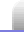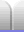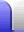# Back-of-the-envelope Calculations

A "back-of-the-envelope" calculation is one that is done very roughly, to give an idea of whether something will work. To do the working any scrap of paper will do, including the back of an envelope; no calculators should be required.

Quantities are often rounded to 1 significant figure. How to combine the available information is often made easy by looking at the units, because unwanted units can be made to cancel out.

Back-of-the-envelope calculations are very much examples of physics in the real world. The calculations are are practical and quick, and often very useful.

### Continuous shower

Let's say I want to heat water for a continuous shower while camping, using a butane stove. Will I be able to heat enough water quickly enough to make real-time heating an option?

• Initial water temperature = 20 °C.
• Final water temperature = 40 °C.
• Change in water temp = 20 °C.
• Specific heat capacity of water at the temperatures we're talking about is 4.18 J/°C/mL; let's call it 4.
• 20 °C * 4 J/°C/mL = 80 J/mL.
• Stove output = 2.4 kW.
• Conversion efficiency = 50%. I'll assume I can easily capture half that heat in the water in real-time.
• Stove effective output = 2.4 kW * 50% = 1.2 kW = 1.2 kJ/s.
• Heating capacity = 1200 J/s / 80 J/mL = 120/8 = 60/4 = 15 mL/s.
• 15 mL/s = 15*60 mL/min = 150*6 = 300*3 = 900 mL/min.

That's not very much. A quick check of my 12 V camp shower gives 3.4 L/min, or 3 L/min throttled back a bit. That's a pretty generous shower, but less than 1 L/min might be a bit stingy.

Conclusion: Almost.

### Deep hole

On 31 May 2013 I came across a hole on the side of a road, and looking down it looked about 6 metres deep. Then I accidentally dropped some mud down the hole, and the mud took longer to reach the bottom than it would in a 6 m deep hole.

Some timing of further dropped objects ensued.

• Average time = 1.6 s.
• s = ½at2 (extremely useful equation of motion)
• Take a = 10 m/s2, acceleration due to gravity.
• s = 5 * (1.6)2 = 5 * 2.56 = 12.8 metres deep.

Conclusion: The hole was twice as deep as it looked!

That made it worth exploring. Since it was late, we lowered a camera with a light attached. That looked interesting (and was quite fun to do) but it didn't give enough information. After abseiling into it the next day (1 June 2013), it became clear that the lesser depth was an optical illusion caused by the hole widening toward the bottom.

(The hole has now been blocked, so I'm the only person who ever abseiled down it.)

### More...

Some more back of the envelope calculations are on the E = mc2 page.Home Astronomy Chemistry Electronics & Computers Mathematics Physics Field Trips Turn on javascript for email link